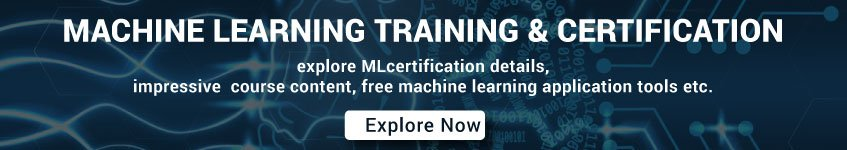# Machine Learning Interview Questions and Answers Set 4

31.       Define What Is Fourier Transform In A Single Sentence?

A process of decomposing generic functions into a superposition of symmetric functions is considered to be a Fourier Transform.

32.       What is the calibration layer in machine learning?

A post-prediction adjustment, typically to account for prediction bias. The adjusted predictions and probabilities should match the distribution of an observed set of labels.

33.       What Is The Difference Between Machine Learning And Data Mining?

Data mining is about working on unstructured data and then extract it to a level where the interesting and unknown patterns are identified.

Machine learning is a process or a study whether it closely relates to design, development of the algorithms that provide an ability to the machines to capacity to learn.

A sophisticated gradient descent algorithm that rescales the gradients of each parameter, effectively giving each parameter an independent learning rate.

35.       Please, State Few Popular Machine Learning Algorithms?

1. Nearest Neighbour

1. Neural Networks

1. Decision Trees etc

1. Support vector machines

36.       What Is The Difference Between Bias And Variance?

Bias: Bias can be defined as a situation where an error has occurred due to the use of assumptions in the learning algorithm.

Variance: Variance is an error caused because of the complexity of the algorithm that is been used to analyze the data.

37.       What is a binary classification in machine learning?

A type of classification task that outputs one of two mutually exclusive classes. For example, a machine learning model that evaluates email messages and outputs either “spam” or “not spam” is a binary classifier.

38.       What is the difference between supervised and unsupervised machine learning?

Supervised learning requires training labeled data. For example, in order to do classification (a supervised learning task), you’ll need to first label the data you’ll use to train the model to classify data into your labeled groups. Unsupervised learning, in contrast, does not require labeling data explicitly.

39.       Explain How We Can Capture The Correlation Between Continuous And Categorical Variable?

Yes, it is possible by using ANCOVA technique. It stands for Analysis of Covariance. It is used to calculate the association between continuous and categorical variables.

40.   What Is Deep Learning?

Deep learning is a process where it is considered to be a subset of the machine learning process.